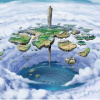novel - Martial Arts

# A Martial Odyssey

## Avidfan

Completed · 978.3K Views

## What is A Martial Odyssey

A Martial Odyssey is a popular web novel written by the author Avidfan, covering HAREM, ROMANCE, CULTIVATION, MARTIAL-ARTS, EPIC ADVENTURE, XIANXIA, WUXIA, HEROIC, EASTERN FANTASY, FANTASY, Martial Arts genres. It's viewed by 978.3K readers with an average rating of 4.86/5 and 14 reviews. The novel is over and can be read all 253 chapters in Webnovel with all rights reserved.

### Synopsis

“It is said that when a martial exponent reached the highest state of divinity of their arts, they be able to transcend into Celestials, overcoming the limitation of life and death. And as Celestials, they have to overcome seven celestial divinity, Genesis, Enlighten, Emotion, Transverse, Seventh Sense, Crisis and Ascend in order to transcend to the Heavens.” When the universal creator Great God Pangu creates the Three Known Realm before giving up the last spark of his life; known as the Mortal Realm, Celestial Realm and the Immortal Realm, he had also left behind a Stellar Sanctuary that will descend upon the Celestial Realm every few hundred years. Those that is able to reach reach the highest level of the Stellar Sanctuary will be able to claim the Pangu Intricate Force and becomes the next Great God Pangu. This is not only a romance that takes place in the martial fraternity but also an ancient tale of love that happens a long time ago… ------------------------------------------------ I post this story from my website to webnovel now. So just enjoy the story without any chapters locked. Please note that the chapters length are actually quite long. You are actually reading 1000 chapters of the typical web novel chapters. So just enjoy the story adventure;)

10 tags

### ratings

• Overall Rate
• Writing Quality
• Updating Stability
• Story Development
• Character Design
• world background

## Reviews14AvidfanAuthorAvidfanjennifer_AugustineLv3jennifer_Augustine

I really like martial oddessy, this is the second time am reading it, I wish the author can write the part 2.l love the mixture of comedy, action and romance in the novel, thanks authorAvidfanAuthorAvidfan

Hope everyone stays safe during this period of cornovirus and be cheerful. Also enjoy the ad-free story. ...................................................................................................................................................................................................................................................................................................................................................................................................................................................................................................................................................................................AvidfanAuthorAvidfan

Shamlessly rating my own works;) :))))))))))))))))))))))))))))))))))))))))))))))))))))))))))))))))))))))))))))))))))))))))))))))))))))))))))))))))))))))))))))))))))))))))))))))))))))))))))))))))))))))))))))))))))))))))))))))))))))))motyLv5moty

i remember reading this over 5 years ago. sssssssssssssppppppppppppppppppaaaaaaaaaaaaaaaaaaccccccccccccccccccccce eeeeeeeeeeeeeeeeeeeeeeeeeeAvidfanAuthorAvidfan

Shamlessly rating my own works;) :))))))))))))))))))))))))))))))))))))))))))))))))))))))))))))))))))))))))))))))))))))))))))))))))))))))))))))))))))))))))))))))))))))))))))))))))))))))))))))))))))))))))))))))))))))))))))))))))))))))